LISEM Classic

LISEM

# Classification

First we can download a sentinel-2 image, which features 12 bands as induvidual jp2 files.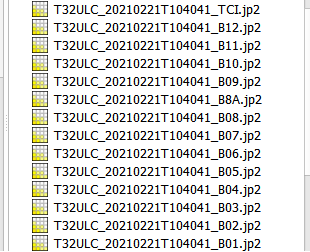These are automatically supported by both the map viewer as well as the scripting language. To cut out an extent of the dataset and store as a single-band GeoTiff file, the following script can be used:

void main()
{
//get the desired bands in a list
array<string> names = GetItemsContaining(GetFilesInDir(),{"B01","B02","B03","B04","B05","B06","B07","B08"});

//set the desired area and create a mask by cutting the second band (which is 10 meter resolution)
Region area = Region(301575,334651,5.72216e+06,5.75079e+06);

//make a map array, and put each band cut and warped to the right resolution (10 meters)
array<Map> bands;
for(int i = 0; i < names.length(); i++)
{
}

//Store result in multi-band tif file
result.tif = bands;
}


Viewing the result.tif file shows the following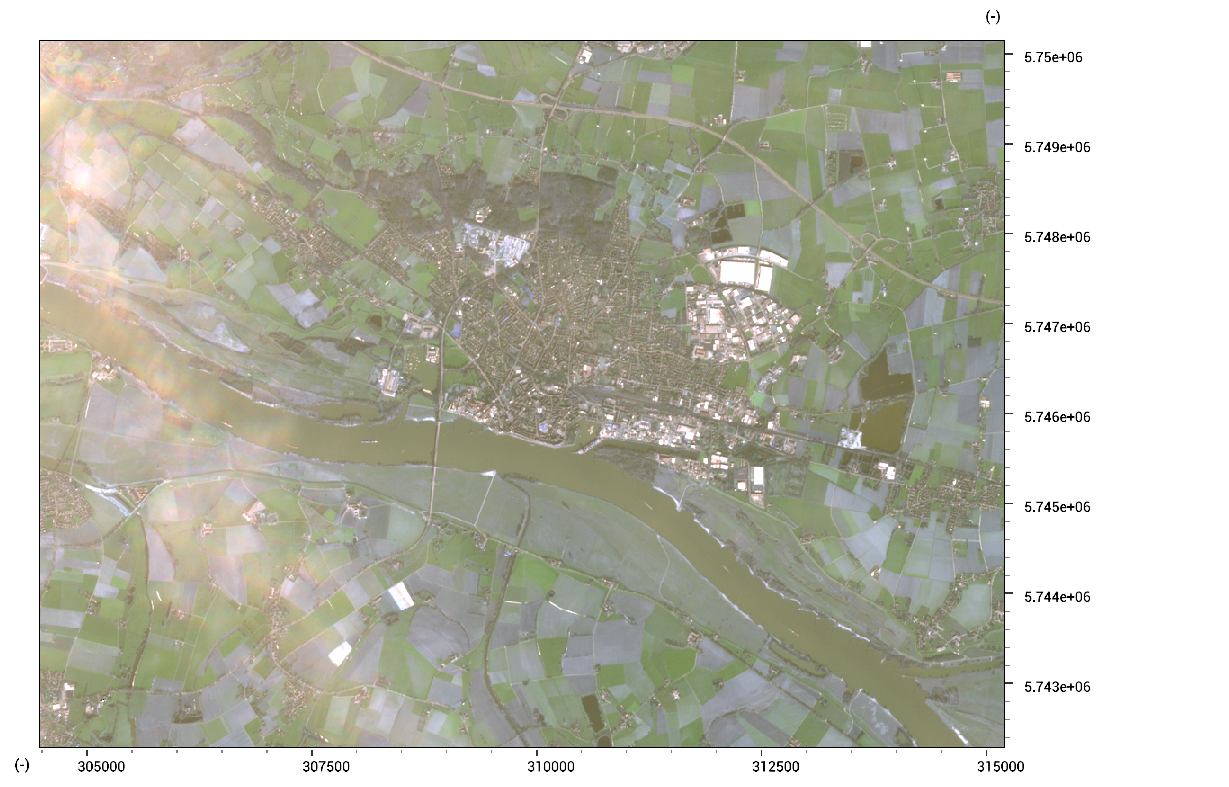Now, a supervised classification can be done. First, create a new empty map:

training.tif = result.tif * 0.0;


Then, use the raster painter, or vector-to-raster conversion to put training data into this map.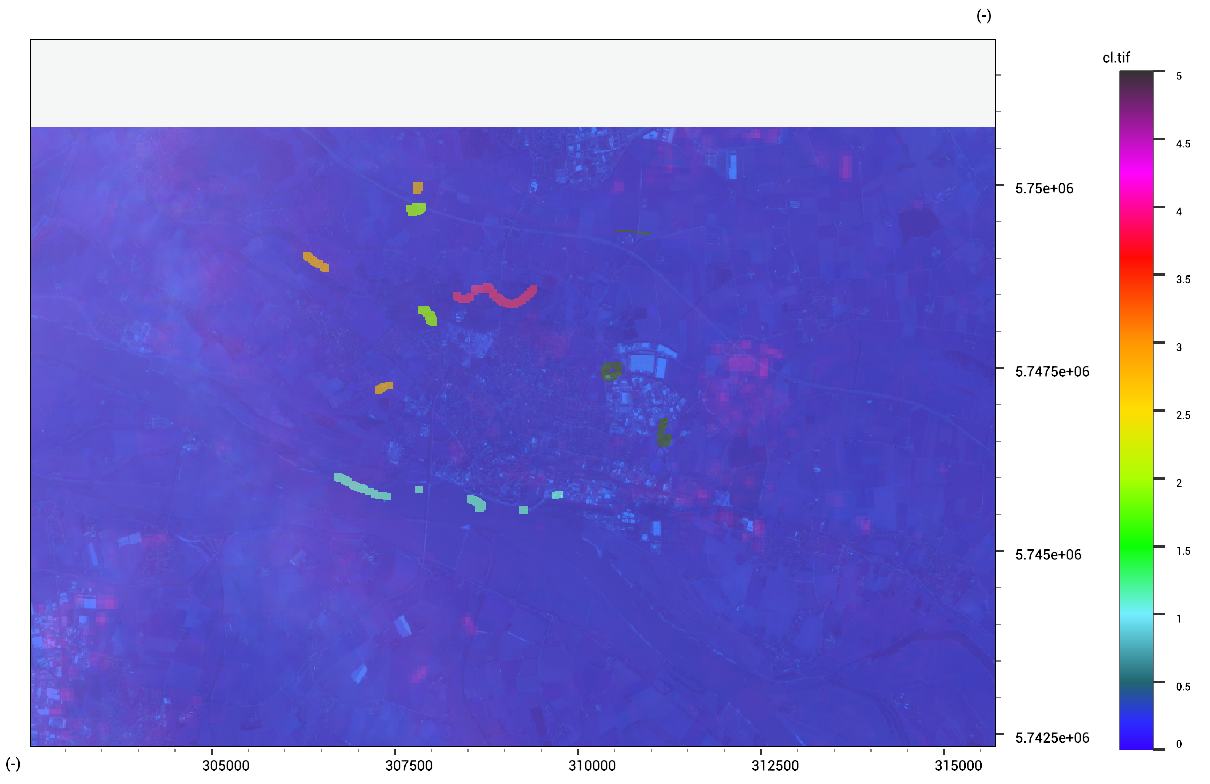Finally, run a Support-Vector-Machine classification algorithm using the following code:

classes.tif = SupervisedClassification(result.tif, training.tif);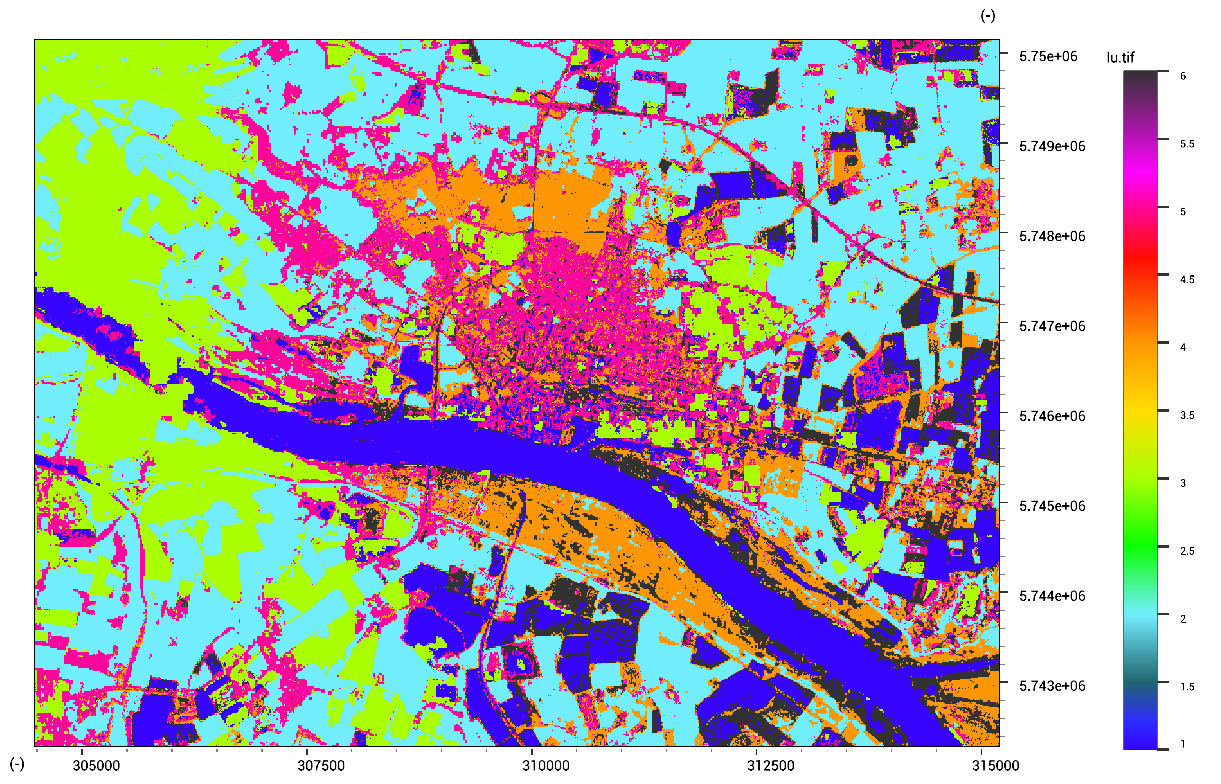In order to remove small regions within the classification, the map can be regularized. This acts similar to WindowMajority, but is a bit faster. We can regularize with a radius of 10 pixels using the following code:

classes_reg.tif = ClassRegularize(classes.tif, 10);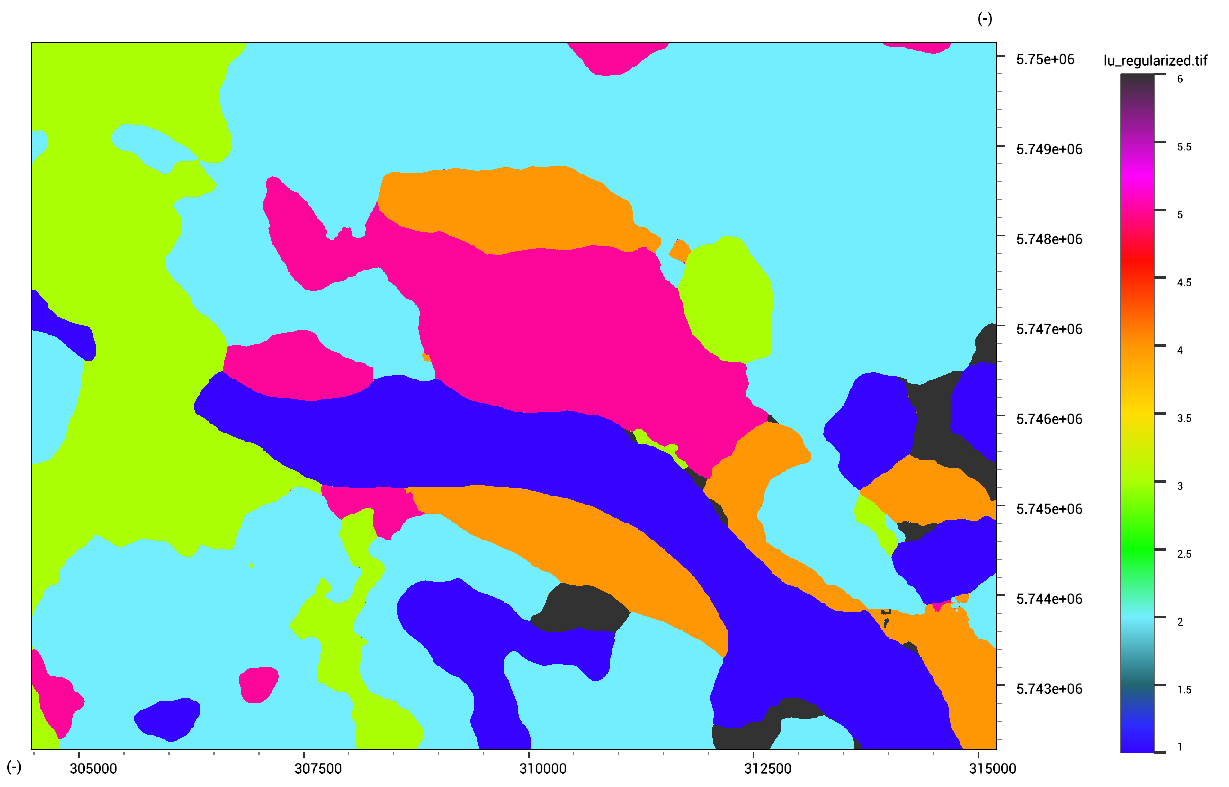An unsupervised classification can be made by specifying the number of classes (5 in our case). It can help to clip the value range of maps, and remove outliers due to clouds.

classes_unsuper.tif = UnSupervisedClassification(result.tif, 5);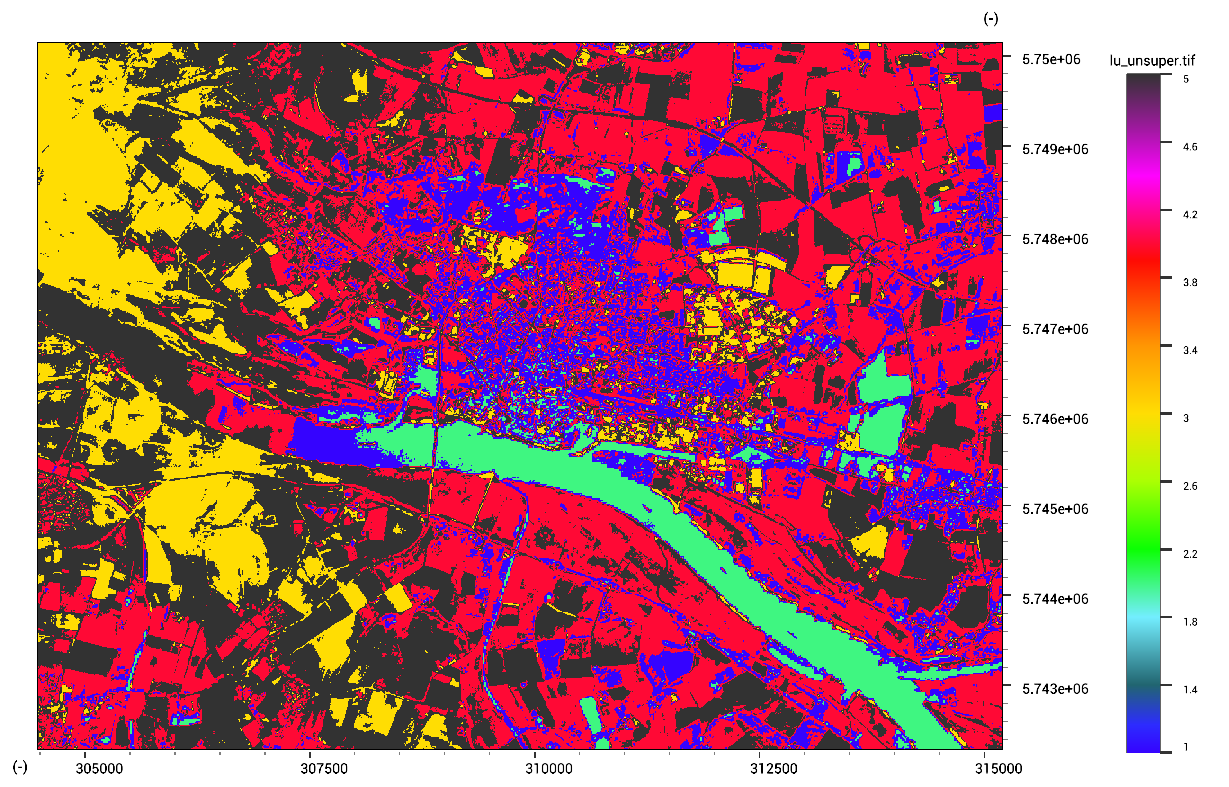We can compare two classified maps by calculating the confusion matrix. This can be done to compare different methods, or compare to ground truth data. Here, to get a confusion matrix between the supervised and unsupervised classification, the following code can be used:

confusionmatrix.tbl = MapConfusionMatrix(classes.tif,classes_unsuper.tif);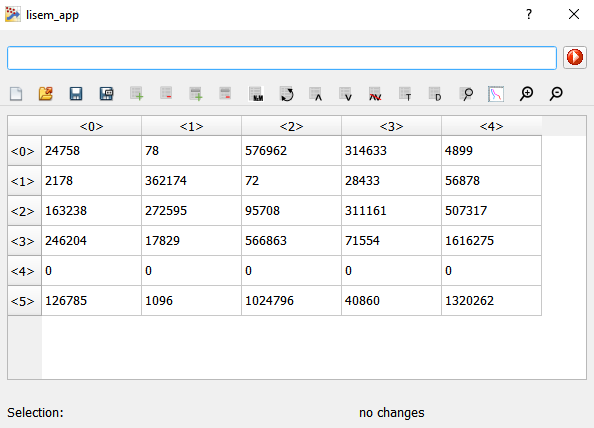Finally, a logistic binary classification can be made, for example for landslide susceptibility.

MathExpression expr = MapLogisticRegression({MapNormalize(slope.map),MapNormalize(dem.map),MapNormalize(pga.map)},training.map);
Print(expr.GetExpression());


The returned expression can be converted to a string by using expr.GetExpression().